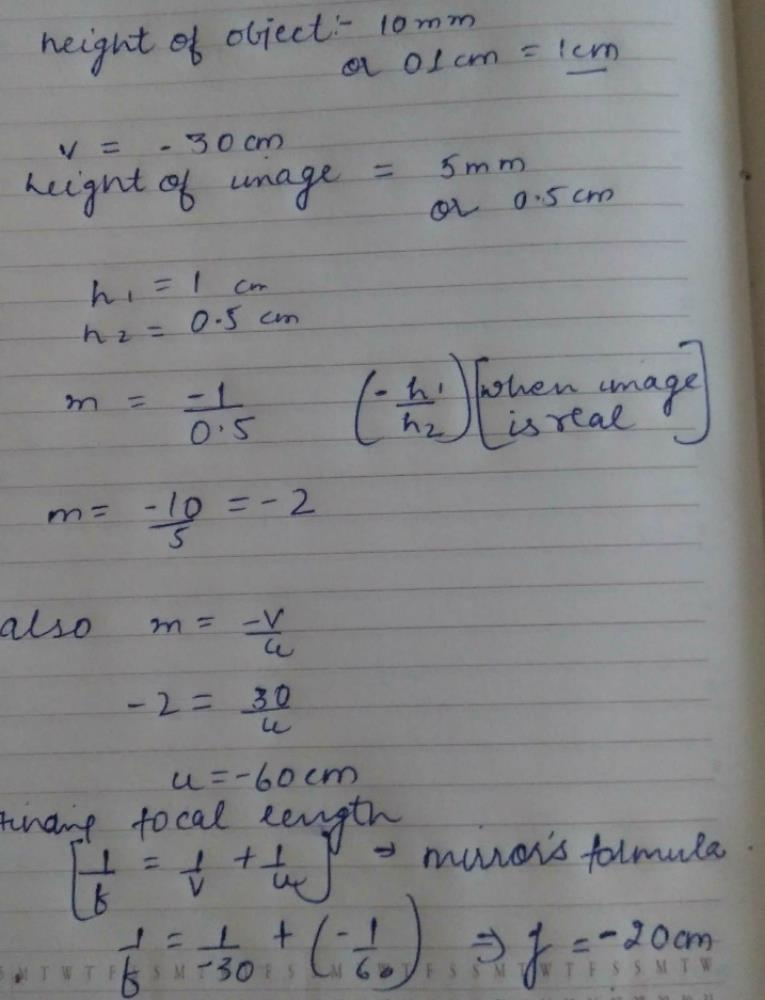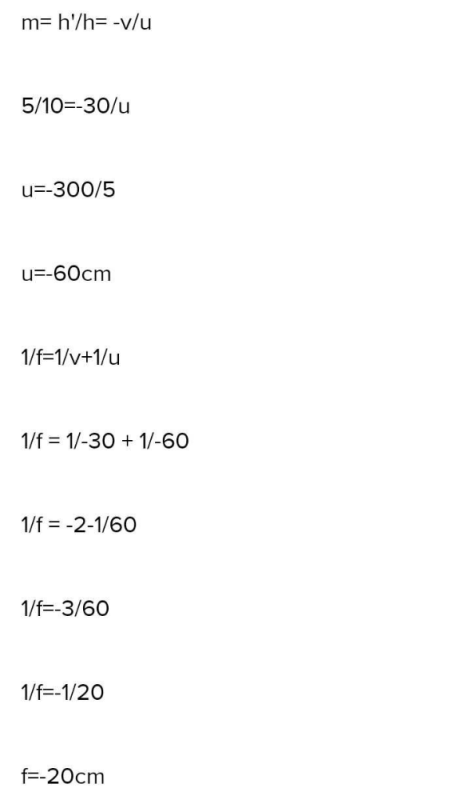Courses

# A 10 mm long awl pin is placed vertically in front of a concave mirror. A 5 mm long image of the awl pin is formed at 30 cm in front of the mirror. The focal length of this mirror isa)- 30 cmb)- 20 cmc)- 40 cmd)- 60 cmCorrect answer is option 'B'. Can you explain this answer? Related Test: Light : Reflection And Refraction - 1

## Class 10 Question

By Azahruddin · Jun 13, 2020 ·Class 10
Poonam Rani answered May 14, 2020Tanishka Gupta answered Jun 13, 2020
H0=10mm hi=5mm v=−30cm
Here  given ,
Now we know
m=−v u =hi ho ⇒u=−vho hi =−30*10 5 =−60 cm  Now using mirror formula  1 f =1 v +1 u =1 −30 +1 −60 =−1 20 Hence f=−20 cm

2

Nirmal Kumar answered Feb 15, 2020
V=-30cm,ho=10mm=1cm,hi=5mm=0.5cm,f=?,we know that-->hi/ho=-v/u,0.5/1=-(-30)/u,u=60cm,by mirror formula-->1/f=1/v-1/u,1/f=1/30-1/(60),1/f=3/(-60),f=-60/3=-20 cm

Likshika Sethi answered Aug 19, 2020

Shiwangi Bansal answered 2 weeks ago
B) -20 cm is the answer

Jerome Job answered 3 weeks ago
Height of the object = 10mm ->1cm
height if the image =( -5mm)->-0.5cm
m=hi/ho, v=(-30cm)
m=( -0.5)/1=(-0.5)
m=(-v/u)
(-0.5)=-(-30)/u
u=(-60cm)
1/v+1/u=1/f
1/-30+(-1/60)=1/f
f=(-20cm)